## Single LED - Series LED's - Parallel LED's

Resistance = (Supply Voltage - LED Voltage) / amperage

## Single LED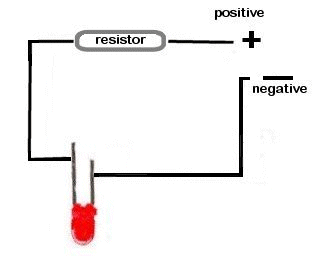Supply Voltage
VOLTS

Voltage Drop Across LED
VOLTS

Desired LED Current
MILLIAMPS

Calculated Limiting Resistor
OHMS
Nearest higher rated 10% resistor

Calculated Resistor Wattage
WATTS
Safe pick is a resistor with
power rating of
WATTS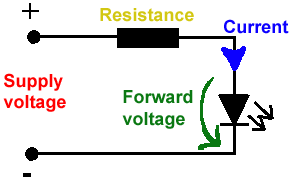## Series LED's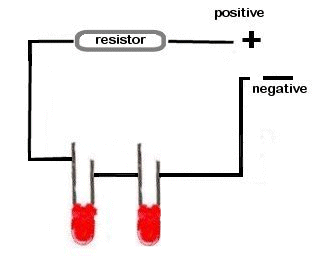Supply Voltage
VOLTS

Voltage Drop Across LED
VOLTS

Desired LED Current
MILLIAMPS
How many leds connected

Calculated Limiting Resistor
OHMS
Nearest higher rated 10% resistor

Calculated Resistor Wattage
WATTS
Safe pick is a resistor with
power rating of
WATTS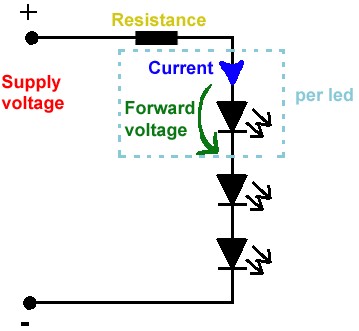## Parallel LED's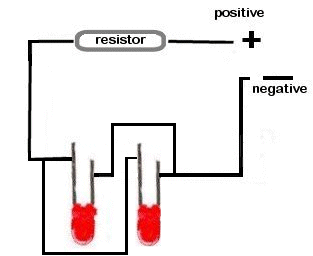Supply Voltage
VOLTS

Voltage Drop Across LED
VOLTS

Desired LED Current
MILLIAMPS

How many leds connected

Calculated Limiting Resistor
OHMS
Nearest higher rated 10% resistor

Calculated Resistor Wattage
WATTS
Safe pick is a resistor with
power rating of
WATTS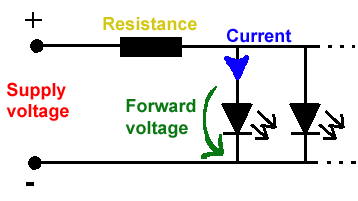Original LED checker script by Nannette Thacker at: http://www.shiningstar.net

Found at Quickar Electronics, Inc

Home